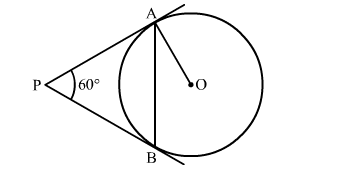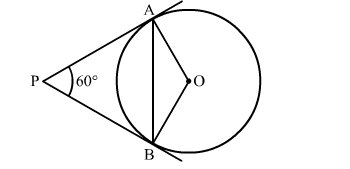# In the given figure, PA and PB are two tangents to the circle with centre O.`
Question:

In the given figure, PA and PB are two tangents to the circle with centre O. If  ∠APB = 60 then ∠OAB is

(a) 15
(b) 30
(c) 60
(d) 90Solution:

Construction: Join OBWe know that the radius and tangent are perperpendular at their point of contact
∵∠OBP = ∠OAP = 90
∠AOB + ∠OBP + ∠APB + ∠OAP = 360            [Angle sum property of a quadrilateral]
⇒ ∠AOB + 90 + 60∘ + 90 = 360
⇒ 240 + ∠AOB = 360
⇒ ∠AOB = 120
Now, In isoceles triangle AOB
∠AOB + ∠OAB + ∠OBA = 180           [Angle sum property of a triangle]
⇒ 120 +  2∠OAB = 180                          [∵∠OAB = ∠OBA ]
⇒ ∠OAB = 30
Hence, the correct answer is option (b).